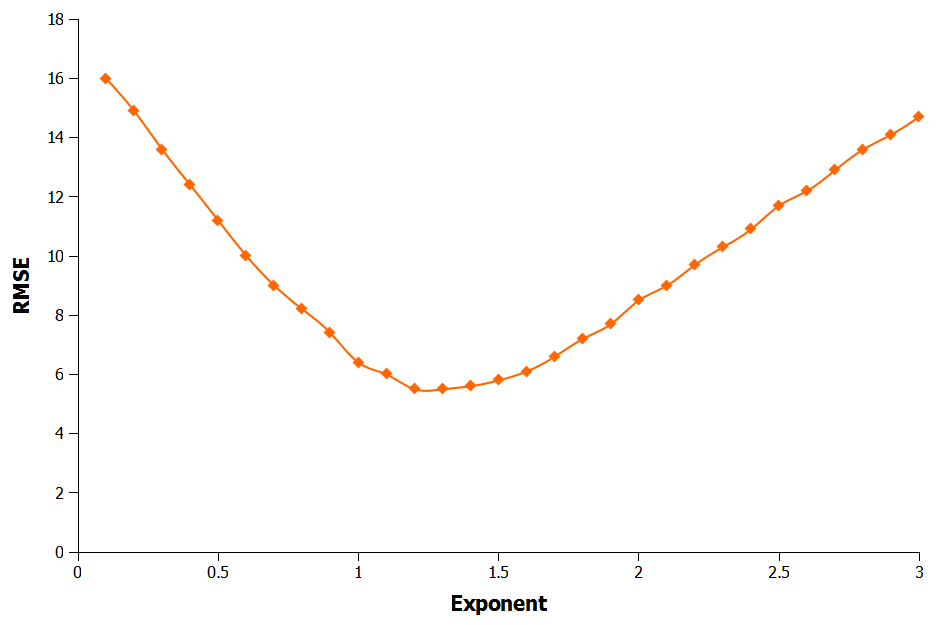# Applying the Pythagorean Expectation to Football: Part One

## Introduction

The Baseball Pythagorean Expectation is a formula originally derived by Bill James to estimate how many games a baseball team could be expected to win over a season based on the number of runs they score and concede (Figure 1). Teams winning fewer games than their Pythagorean prediction are considered to have been unlucky while those outperforming the prediction are thought to have had luck on their side.

$$wins = runs scored^2 / (runs scored^2 + runs allowed^2)$$

Figure 1: The Baseball Pythagorean Expectation

The formula works well for baseball, giving predictions generally within three games of what actually happens. The Pythagorean expectation has also been applied successfully to other sports, including American football and basketball. However, so far the equation has not worked particularly well for predicting football matches.

Table 1 shows goals scored and conceded in the English Premier League (EPL) during the 2011–2012 season, along with the actual points and Pythagorean predicted points. Looking at the difference between predicted and actual points it is clear that the Pythagorean expectation is over-predicting at the top of the table and under-predicting at the bottom.

 Team GF GA Pts Pythag Pts Manchester City 93 29 89 104 Manchester United 89 33 89 100 Arsenal 74 49 70 79 Tottenham Hotspur 66 41 69 82 Newcastle United 56 51 65 62 Chelsea 65 46 64 76 Everton 50 40 56 70 Liverpool 47 40 52 66 Fulham 48 51 52 54 West Bromwich Albion 45 52 47 49 Swansea City 44 51 47 49 Norwich City 52 66 47 44 Sunderland 45 46 45 56 Stoke City 36 53 45 36 Wigan Athletic 42 62 43 36 Aston Villa 37 53 38 37 Queens Park Rangers 43 66 37 34 Bolton Wanderers 46 77 36 30 Blackburn Rovers 48 78 31 31 Wolverhampton Wanderers 40 82 25 22 RMSE 8.4

Table 1: Pythagorean Expectation for the EPL 2011–2012

We can quantify this error by calculating the root-mean-square error (RMSE). This technique basically squares the difference between the predicted and actual points and then takes the square root of the average. It sounds complicated but all the squares and square roots do is make all the numbers positive. Imagine if we predicted just two values and were -10 points out for the first and +10 points out on the second. If we just averaged these two numbers then the average error would be zero, making it look like our prediction was perfect when it obviously was not. Instead, if we square the numbers first and then take the square root of the average we get the correct error of ten points. Doing this calculation for Table 1 gives us a RMSE of 8.4 points meaning that on average the Pythagorean expectation was eight points out for the 2011–2012 season.

The more accurate the predictions are then the lower the RMSE will be. One way to improve the prediction is to alter the exponent used in the equation. In other words, instead of raising goals scored and conceded to the power of two we use different values. Figure 2 shows what happens to the RMSE as the exponent is changed from 0.1–3. Looking at the chart, the RMSE is lowest using an exponent of 1.35, giving an average error of 5.75, nearly three points lower than before.Figure 2: Effect of Altering Exponent on RMSE

The next logical step to improve the prediction further is to try using a different exponent for each part of the equation. This makes the formula harder to optimize but by applying a technique called least squares to it we come up with optimal exponents of 1.39, 1.43 and 0.98. Unfortunately this has little effect on the RMSE though, reducing it just 0.1 to 5.65 points.

So far the predictions are still nearly six points out but in part two of this article I will discuss why the error is high and show how to improve it further to increase the accuracy of the predictions.

## Get In Touch!

Submit your comments below, and feel free to format them using MarkDown if you want. Comments typically take upto 24 hours to appear on the site and be answered so please be patient.

Thanks!